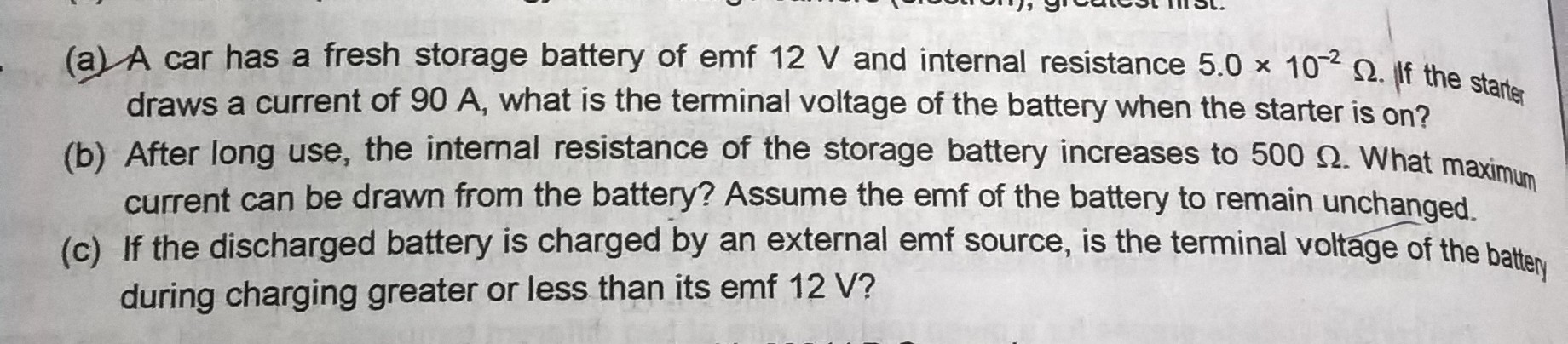# will be very kind if reply for the answer myself yogesh from village tilpat in Faridabad4 years ago

we know that

the relation between terminal voltage (V) and emf (E) of a battery is given as

V = E - Ir

here,

E = 12 volts

I = 90 amps

r = 0.05 ohms

so,

V = 12 - 90x0.05

thus, terminal voltage

V = 7.5 volts

now,

the maximum current will be calculated for a short circuit when voltage is zero

so, if V = 0

E = Ir

or

Imax = E/r

here,

r = 500 ohms

so,

Imax = 12/500

thus, maximum current

Imax = 0.024 amps